NEET  >  Short & Long Answer Questions: Gravitation - 2

# Short & Long Answer Questions: Gravitation - 2 - Physics Class 11 - NEET

Q.51 The escape velocity for a satellite is 11.2 kms−1. If the satellite is launched at an angle of 60 with the vertical, what will be the escape velocity?

Answer: 11.2kms−1, because escape velocity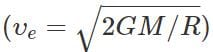does not depend on the angle of projection.
Q.52 An astronaut, while revolving in a circular orbit happens to throw a spoon outside. Will the spoon reach the surface of the earth?

Answer: The spoon will continue to move in the same circular orbit, and chase the astronaut. It will never reach the surface of the earth.
Q.53 An artificial satellite revolves around the earth without using any fuel. On the other hand, an aeroplane requires fuel to fly. Why?

Answer: Air is essential for an aeroplane to fly. To overcome the resistive forces of air, aeroplane requires fuel. The satellite orbits at a much higher height where air resistance is negligible and so it needs no fuel.
Q.54 Why are space rockets usually launched from west to east in the equatorial plane?

Answer: Due to rotation of the earth about its polar axis, every particle on the earth has a linear velocity directed from west to east. This velocity (υ=Rω)) is maximum at the equator. When a rocket is launched from west to east, this maximum velocity gets added to the launching velocity, so the launching becomes easier.
Q.55 A satellite of small mass burns during its descent and not during ascent. Why?

Answer: The speed of the satellite during descent is much larger than that during its ascent. As the air resistance is directly proportional to velocity, so heat produced during descent is very large and the satellite burns up.

Q.56 Is it possible to place an artificial satellite in an orbit such that it is always visible over New Delhi?

Answer: No. A. satellite remains always visible only if it revolves in the equatorial plane with a period of revolution equal to that of the earth. New Delhi does not lie in the region of equatorial plane.
Q.57 The astronauts in a satellite orbiting the earth feel weightlessness. Does the weightlessness depend upon the distance of the satellite from the earth? Give reason.

Answer: No. The gravitational acceleration of the astronaut relative to the satellite is zero, whatever be the distance of the satellite from the earth.
Q.58 Can we determine the gravitational mass of a body inside an artificial satellite?
No, because both the body and the artificial satellite are in a state of free fall.
Q.59 Why an astronaut in an orbiting space-craft is not in zero gravity although weightless?

Answer: The astronaut in the orbiting spacecraft is still in the gravitational field of the earth and experiences appreciable gravity. However, the gravity is used up in providing the necessary centripetal force.
Q.60 Why does an astronaut in spacecraft feel weightlessness?

Answer: This is because the orbiting spacecraft along with the astronaut is in a state of free fall towards the earth.
Q.61 Two identical geostationary satellites are moving with equal speeds in the same orbit but their sense of rotation brings them on a collision course. What will happen to the debris?

Answer: The collision between the two satellites is inelastic. They stick together.                 mυ+(−mυ)=(m+m)V or V=0  The kinetic energy of the debris in its orbit will be zero. Due to gravity, it falls to the surface of the earth and in the course of its journey, it may get burnt up.
Q.62 The linear speed of a rocket is not constant in its orbit. Comment.

Answer: The areal velocity of a planet around the sun is constant but its linear speed continuously changes. The linear speed of the planet is large when it passes close to the sun and is small when the planet is away from the sun.
Q.63 The largest and shortest distances of the earth from the sun are r1 and rrespectively. What is the distance from the sun when it is perpendicular to the major axis of the orbit from the sun?

Answer: Let R be the distance of the earth from the sun in the position perpendicular to the major axis of its elliptical orbit. Using the property of an ellipse, we can write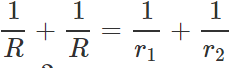or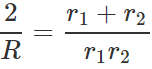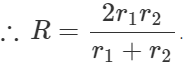Q.64 Why does the weight of a body become zero at the centre of the earth?

Answer: As we go inside the earth, the value of attracting mass M decreases and its value becomes zero at the centre of the earth. Consequently, a body at the centre of the earth feels no gravitational attraction or its weight becomes zero at the centre of the earth.
Q.65 Imagine a spacecraft going from the earth to the moon. How does its weight vary as it goes from the earth to the moon?

Answer: (i) As the spacecraft moves from the earth towards the moon, its weight decreases.

(ii) The weight of the spacecraft becomes zero at the null point where the gravitational forces of the earth and the moon are equal and opposite.

(iii) Again the weight increases, becoming mg/6 on the moon's surface.
Q.66 The artificial satellite does not have any fuel, but even then it remains in its orbit around the earth. Why.

Answer: The centripetal force necessary for orbital motion of the satellite is provided by the force of gravitation exerted by the earth on the satellite. So the satellite does not need any fuel for its orbital motion.
Q.67 According to Albert Einstein, no particle can have velocity equal to or greater than that of light. Hence what may be the value of escape velocity from the surface of a 'Black hole' such that it holds everything to itself?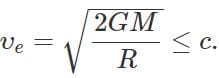Q.68 Answer the following : (a) Among the known types of forces in nature, the gravitational force is the weakest. Why then does it play a dominant role for motion of bodies on the terrestrial, astronomical and cosmological scale? (b) Do the forces of friction and other contact forces arise due to gravitational attraction? If not, what is the origin of these forces?

Answer: (a) The strong nuclear forces operate only over a range of distance of the order of 10−14 to 10−15m.. The forces involved in weak interactions operate only during radioactive decay. Electrical forces are stronger than gravitational forces for a given distance, but they can be attractive as well as repulsive unlike gravitational force, which is always attractive. As a result, the forces between massive neutral bodies are predominantly gravitational.

(b) No, the forces of friction and other contact forces have electromagnetic origin.
Q.69 Choose the correct alternatives : (i) If the gravitational potential energy of two mass points infinite distance away is taken to be zero, the gravitational potential energy of a galaxy is (positive/negative/zero). (ii) The universe on the large scale is shaped by (gravitational/electromagnetic) forces, on the nuclear scale by (gravitational/ electromagnetic/ strong nuclear) forces.

Answer: (i) The gravitational potential energy of a galaxy is negative because it is a bound system.

(ii) The universe on the large scale is shaped by gravitational forces, on the atomic scale by electromagnetic forces and on the nuclear scale by strong nuclear forces.
Q.70 What is the difference between inertial mass and gravitational mass of a body?

Answer: The inertial mass of a body is a measure of its inertia and is given by the ratio of the external force applied on it to the acceleration produced in it. The gravitational mass of a body, on the other hand, is a measure of the gravitational pull acting on it due to the earth. The gravitational mass is measured by a common balance.

Q.71 Which of the following observations point to the equivalence of inertial and gravitational mass: (a) Two spheres of different masses dropped from the top of a long evacuated tube reach the bottom of the tube at the same time. (b) The time-period of a simple pendulum is independent of its mass. (c) The gravitational force on a particle inside a hollow isolated sphere is zero. (d) For a man in closed cabin that is falling freely under gravity, gravity 'disappears'. (e) An astronaut inside a spaceship orbiting around the Earth feels weightless. (f) Planets orbiting around the sun obey Kepler's Third Law (approximately). (g) The gravitational force on a body due to the Earth is equal and opposite to the gravitational force on the Earth due to the body.

Answer: The observations (a), (b), (d (e) and (f) point to the equivalence of gravitational masses, because in these situations the bodies are in motion. The observations (c) and (g) do not establish the equivalence of inertial and gravitational masses because the bodies are not in the state of motion.
Q.72 A body is taken from the centre of the earth to the moon. What will be the changes in the weight of the body?

Answer: (i) At the centre of the earth, the weight of the body is zero (g=0).

(ii)As the body is moved from centre to the earth surface, its weight increases (due to increasing)

(iii) As the body is moved from earth's surface towards the moon, the weight decreases (due to the decreasing), becomes zero at the point where the gravitational forces of the earth and moon are equal and opposite.

(iv) Again the weight increases, becoming on the moon's surface.
Q.73 Mention the conditions under which the weight of a person can become zero.

Answer:  The weight of a person can become zero under the following conditions:

(i) When the person is at the centre of the earth (as g =0 at the centre of the earth).

(ii) When the person is at the null points in space (At these points, the gravitational forces due to different masses cancel out).

(iii) When the person is standing in a freely falling lift.

(iv) When the person is inside a spacecraft which is orbiting around the earth.
Q.74 How will the value of g be affected if  (i) the rotation of the earth stops (ii) the rotational speed of the earth is doubled (iii) the rotational speed of the earth is increased to seventeen times its present value?

Answer: (i) If the rotation of the earth stops, no centrifugal force will act on the bodies lying on it. The value of g increases maximum at the equator. As no centrifugal force acts on body at poles, so the value g is not affected.

(ii) If the rotational speed of the earth is doubled, the centrifugal force on the bodies increases. The value of g decreases maximum at the equator and is not affected at poles. (iii) If the rotational speed of the earth is increased to seventeen times its present value, the value of g at the equator will become zero. At the poles, the value of g remains unchanged.
Q.75 When a satellite moves to a lower orbit in the atmosphere of the earth, it becomes hot. This indicates that there is some dissipation in its mechanical energy. But the satellite spirals down towards the earth with an increasing speed, why?

Answer: For a satellite orbiting the earth, Total energy =− Kinetic energy i.e., E=−K When the satellite enters the atmosphere of the earth, it dissipates its mechanical energy (which is negative) against atmospheric friction. Energy E becomes more negative. As a result, the kinetic energy of the satellite increases and hence its speed increases. But its orbital speed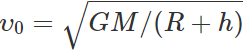can increase only if its height h becomes smaller. Hence the satellite moves to a lower orbit with an increased speed. Thus due to the atmospheric friction, the satellite spirals down towards the earth with increasing speed and ultimately bums out in the lower dense atmosphere.
Q.76 What are the conditions under which a rocket fired from the earth becomes a satellite of the earth and orbits in a circle?

Answer: (i) First the rocket should be given a sufficient vertical velocity so that it reaches a height at which it is supposed to revolve around the earth.

(ii) At this height, the rocket must be given a horizontal orbital velocity given by: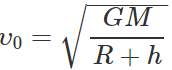(iii) The air resistance should be negligible at the height of its orbit.
Q.77 What would happen if the force of gravity were to disappear suddenly?

Answer: If the force of gravity suddenly disappears, then

(i) All bodies will lose weight.

(ii) We would be thrown away from the earth due to the centrifugal force.

(iii) Eating, drinking and in fact all operations would become impossible.

(iv) Motion of satellites around the planets and the motion of planets around the sun would cease.
Q.78 The time period of the satellite of the earth is 5 hours. If the separation between the earth and the satellite is increased to 4 times the previous value, then what will be the new time period of the satellite?

Answer: According to Kepler's law of periods,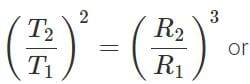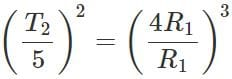or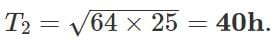Q.79 Why is the weight of a body at the poles more than the weight at the equator? Explain.

Answer:  As g=GM/R2 and the value of R at the poles is less than that at the equator, so g at poles is greater that g at the equator. Now, as  gp>ge, hence mgp>mgi.e., the weight of a body at the poles is more than the weight at the equator.
Q.80 Why does the earth impart the same acceleration to all bodies?

Answer: The force of gravitation exerted by the earth on a body of mass m is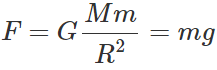Acceleration imparted to the body,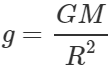Clearly, g does not depend on m. Hence the earth imparts same acceleration to all bodies.
Q.81 If suddenly the gravitational force of attraction between the earth and a satellite revolving around it becomes zero, what will happen to the satellite?

Answer: If the gravitational force of the earth suddenly becomes zero, the satellite will stop revolving around the earth and it will move in a direction tangential to its original orbit with a speed with which it was revolving around the earth.

The document Short & Long Answer Questions: Gravitation - 2 | Physics Class 11 - NEET is a part of the NEET Course Physics Class 11.
All you need of NEET at this link: NEET

## FAQs on Short & Long Answer Questions: Gravitation - 2 - Physics Class 11 - NEET

 1. What is the law of gravitation?Ans. The law of gravitation states that every particle in the universe attracts every other particle with a force that is directly proportional to the product of their masses and inversely proportional to the square of the distance between their centers.
 2. How does the law of gravitation explain the motion of planets?Ans. The law of gravitation explains the motion of planets by stating that the gravitational force between the Sun and each planet is responsible for their orbital motion. The force acts as a centripetal force, keeping the planets in their elliptical paths around the Sun.
 3. What is the difference between mass and weight?Ans. Mass is the amount of matter present in an object and is a scalar quantity, while weight is the force experienced by an object due to gravity and is a vector quantity. Mass is measured in kilograms, while weight is measured in newtons.
 4. How does distance affect the gravitational force between two objects?Ans. According to the law of gravitation, the gravitational force between two objects decreases as the distance between them increases. It follows an inverse square relationship, which means that doubling the distance between two objects will result in a quarter of the gravitational force.
 5. Can the law of gravitation be applied to objects on Earth's surface?Ans. Yes, the law of gravitation can be applied to objects on Earth's surface. The force of gravity between any two objects, such as a person and the Earth, can be calculated using the law of gravitation. However, in most cases on Earth's surface, the force of gravity is dominated by the Earth's mass and can be approximated using the equation for weight (W = mg), where m is the mass of the object and g is the acceleration due to gravity.

## Physics Class 11

130 videos|483 docs|210 tests

## Physics Class 11

130 videos|483 docs|210 tests

### How to Prepare for NEET

Read our guide to prepare for NEET which is created by Toppers & the best Teachers
Signup to see your scores go up within 7 days! Learn & Practice with 1000+ FREE Notes, Videos & Tests.
10M+ students study on EduRev
Track your progress, build streaks, highlight & save important lessons and more!(Scan QR code)
Related Searches

,

,

,

,

,

,

,

,

,

,

,

,

,

,

,

,

,

,

,

,

,

;